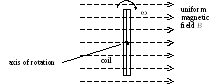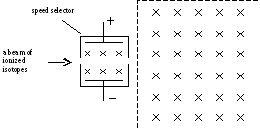# 02 al physics/Essay/P. Hong kong advanced level examination

 02 AL Physics/Essay/P. HONG KONG ADVANCED LEVEL EXAMINATION AL PHYSICS 2002 Essay Type Question 1. (a) With the aid of examples, state the magnitude and direction of the resultant force acting on an object that performs (i) projectile motion, (ii) uniform circular motion, and (iii) simple harmonic motion. How does the resultant force bring about the motion in each case? (8 marks) (b) (i) A boy starts to jump vertically from a horizontal ground. Identify all action and reaction pairs involved. Account for his initial acceleration. (ii) Use the concept of action and reaction to explain the launching of rockets. (5 marks) (c) Consider the head-on collision of an -particle with an isolated gold nucleus that is stationary initially. Explain whether or not the linear momentum of the system is conserved. Describe the energy change during the collision process. (Assume that no excitation of the gold nucleus occurs.) (3 marks) 2. (a) (i) With the aid of a diagram, describe an experiment to demonstrate the interference of a 3 cm microwave using one microwave transmitter. State the appropriate spatial arrangement of the apparatus and briefly explain the experimental result. (ii) State and explain a practical application involving the interference of waves. (6 marks) (b) Large fishing boats are usually equipped with radars and sonars. Describe the working principles of each equipment and state their respective functions. (4 marks) (c) Tidal energy is a renewable energy source. (i) Why is tidal energy classified as ‘renewable’? Describe the principles of a method of harnessing it. (ii) Discuss the factors that need to be considered in harnessing tidal energy near the mouth of a river. (6 marks) 3. (a) (i) Explain the meaning of forced oscillation and resonance. State the phase relationship, in the case of resonance, between the driving force and the displacement of the system that is being driven. (ii) Sketch graphs to show how the amplitude of forced oscillation depends on the driving frequency when the damping is (I) light and (II) heavy. State and explain TWO considerations in the design of the suspension of a car by referring to the graphs sketched. (8 marks) (b) Describe a practical example of resonance which is (i) acoustic and (ii) electrical. For each example, discuss the factor(s) affecting the resonant frequency. (5 marks) (c) Briefly explain how a laser can produce an intense, monochromatic beam. (There is no need to describe the setting up of an inverted population.) (3 marks) 4. (a) (i) Describe the principles of an experiment that can reveal the sign of the charge carriers in a current-carrying conductor. (ii) Describe and explain, with the aid of graphs, the relation between the current and the applied voltage for (I) a tungsten filament lamp in daily use and (II) a semiconductor diode. Explain in each case any deviation from Ohm’s law. (9 marks) (b) A rectangular coil of N turns, each of area A, is placed with its plane perpendicular to a uniform magnetic field of flux density B as shown. At time t = 0 s, the coil is rotated in a clockwise direction with a uniform angular speed .(i) Derive an expression for the variation of the induced e.m.f. in the coil with time. Sketch a graph to show its variation within one cycle and indicate when the plane of the coil is parallel to the magnetic field. (ii) In an actual a.c. generator the coil is wound around a soft iron cylinder, which is laminated. State and explain TWO advantages of such a design. (7 marks) 5. (a) Give the meaning of the terms atomic number, mass number and isotopes. (2 marks) (b) You are given some isotopes of a certain element. The isotopes are ionized so that they can carry the same charge Q, and enter a speed selector as shown. Only those isotopes with a definite speed can pass straight through the mutually perpendicular uniform electric and magnetic fields in the speed selector. The whole set-up is in a vacuum environment.(i) Explain how the speed selector works. (ii) Describe and explain how the isotopes can be distinguished experimentally by directing the emerging beam of ionized isotopes into a uniform magnetic field. (7 marks) (c) (i) Give THREE sources of background radiation. (ii) Describe an experiment to show that only -particles and -rays are emitted from a given radioactive source. Account for the experimental result. (7 marks) - End of Paper -Download 28.95 Kb.Share with your friends: